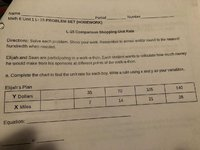# Elijah's Plan: Math

#### harpazo

##### Full Member
Hello friends. My brother texted this question to me for help. As you all know, I'm going through a rough, personal time in my life and not in the math frame of mind. For this question, I need to complete the chart and write an equation. Below is my work in terms of the equation.

Elijah's Plan Equation

Select two points from the chart.

(7,35)

(14,70)

Find the slope.

m = 70 - 35 ÷ 7

m = 35 ÷ 7

m = 5

Use the point-slope formula.

y - 35 = 5(x - 7)

y = 5x - 35 + 35

y = 5x

Correct?

If correct, how is the chart then completed?#### Harry_the_cat

##### Senior Member
Hello friends. My brother texted this question to me for help. As you all know, I'm going through a rough, personal time in my life and not in the math frame of mind. For this question, I need to complete the chart and write an equation. Below is my work in terms of the equation.

Elijah's Plan Equation

Select two points from the chart.

(7,35)

(14,70)

Find the slope.

m = (70 - 35) ÷ 7 Brackets are very important here.

m = 35 ÷ 7

m = 5

Use the point-slope formula.

y - 35 = 5(x - 7)

y = 5x - 35 + 35

y = 5x

Correct? Yes but you can easily check this for yourself. Does every y in the table equal 5 times x?

If correct, how is the chart then completed? Unit rate = ?? dollars per 1 mile

View attachment 14092

#### harpazo

##### Full Member
Yes, every y in the table does equal 5 times x. I do not understand the rest. Is it 7 dollars y for the first mile x?

#### Harry_the_cat

##### Senior Member
Yes, every y in the table does equal 5 times x. Good then your equation must be correct!

I do not understand the rest. Is it 7 dollars y for the first mile x?

#### harpazo

##### Full Member
The unit rate is in \$/mile. ie How much does he get if he walks 1 mile? (in other words if x=1 what is y? Use you equation if you have to.
If he walks 1 mile, he gets 5 dollars. I need to complete the table. Is the table complete by stating y dollars = 5 when he walks 1 mile represented by x here?

#### Harry_the_cat

##### Senior Member
Yes so that's the rate: 5 dollars per mile.

•harpazo

#### harpazo

##### Full Member
Yes so that's the rate: 5 dollars per mile.
To complete the table, we need:

y dollars = 5
x mile = 1

Yes?

Can you help me answer parts b and c for the other question posted? I truly would appreciate your help.

#### Harry_the_cat

##### Senior Member
To complete the table, we need:

y dollars = 5
x mile = 1

Yes?

Can you help me answer parts b and c for the other question posted? I truly would appreciate your help.
Yeah it seems that they are asking for the unit rate to complete the table A bit of a silly question.
10 and 2 also fits the table, 15 and 3, 500 and 100 but I think they want 5 and 1.

•harpazo

#### harpazo

##### Full Member
Yeah it seems that they are asking for the unit rate to complete the table A bit of a silly question.
10 and 2 also fits the table, 15 and 3, 500 and 100 but I think they want 5 and 1.
You are great. Can you check my work for the other question. I am having trouble finding parts b and c.

#### Otis

##### Senior Member
… Find the slope.

m = 70 - 35 ÷ 7 …
Hi. In addition to the missing grouping symbols that Harry mentioned, here's another tip. The slope calculation above is okay for scratch paper, but, if it were being turned in as work shown instead, then we should write out the entire formula:

m = (70 - 35)/(14 - 7)

Doing this helps students call to mind that slope is [the change in y] over [the change in x].#### harpazo

##### Full Member
Thank you everyone.